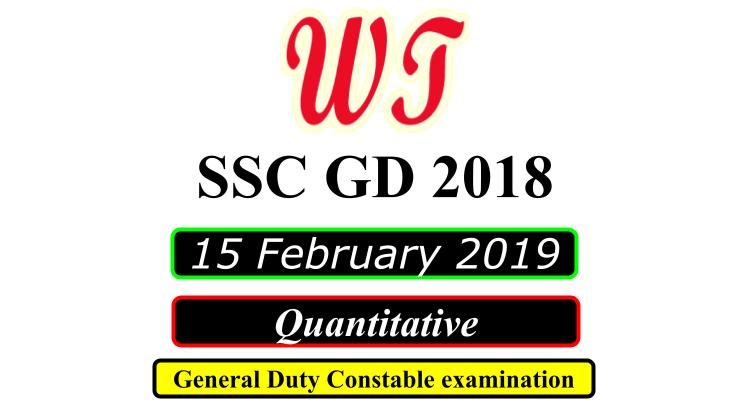Type Here to Get Search Results !

Download SSC (Staff Selection Commission) GD (General Duty), 15 February 2019 ( Math )Quantitative Questions PDF With Answers.

Table of Content

Table of Content (toc)SSC GD 15 February 2019 Quantitative Questions PDF Download Free

### SSC GD 15 Feb 2019 Shift 1 Math Questions

Instructions
For the following questions answer them individually
Q1:-A sum of ₹ 10000 is lent on simple interest at the rate of 15% per annum. What is the difference between Simple interest for 6 years and the simple interest for 2 years?
A.    ₹4000
B.    ₹8000
C.    ₹6000
D.     ₹4500
Q2:-What is the mean of the given data? 2, 3, 4, 5, 6, 7, 8, 9
A.    4
B.    4.5
C.    5.5
D.    5
Q3:-A train is moving with a uniform speed. Train crosses a bridge of length 243 meters in 30 seconds and a bridge of length 343 meters in 36 seconds. What is the speed of the train?
A.    60 km/hr
B.    72 km/hr
C.    64 km/hr
D.    65 km/hr
Q4:-The area of a square and a rectangle are equal. The length of the rectangle is greater than the side of square by 9 cm and its breadth is less than the side of square by 6 cm. What will be the perimeter of the rectangle?
A.    84 cm
B.    74 cm
C.    78 cm
D.    76 cm
Q5:-A car covers a certain distance at a speed of 54 km/hr in 7 hours. What should be the speed of car to cover the same distance in 6 hours?
A.    72 km/hr
B.    56 km/hr
C.    63 km/hr
D.    54 km/hr

### SSC GD 15 Feb 2019 Shift 2 Math Questions

Instructions
For the following questions answer them individually
Q1:-A shopkeeper sells an article at a certain price. What is his gain or loss percent, if by selling it at 75% of that price, he loses 10%?
A.    Gain, 20%
B.    Gain, 25%
C.    Loss, 20%
D.    Loss, 25%
Q2:-A man can row downstream a distance of 9 km in 2 hours and takes 6 hours while returning to the starting point. What is the speed (in km/h) of the stream?
A.    2
B.    2.5
C.    1.5
D.    3
Q3:-The average weight of a certain number of students in a group is 54 kg. if 12 students of average weight 52 kg join the group, then the average weight of all the students in the group decreases by 750 g. What was the number of students, initially in the group?
A.    18
B.    24
C.    15
D.    20
Q6:-The ratio of ages of A and B, 4 years ago, was 5 : 7. The ratio of their ages after 4 years from now will be 7 : 9. What will be the ratio of ages of A and B after 12 years from now?
A.    8 : 13
B.    13 : 17
C.    9 : 11
D.    15 : 19
Q9:-Let x be the least number which when divided by 12, 15, 18, 20 and 27, the remainder in each case is 2, but x is divisible by 23. If x is divided by the sum of its digits then the quotient is:
A.    196
B.    149
C.    193
D.    199

### SSC GD 15 Feb 2019 Shift 3 Math Questions

Q4:-If A is 75% more than B, C is 40% less than D and D is 20% more than A, then which one of the following is true?
A.    63C = 50B
B.    25B = 21C
C.    21B = 25C
D.    50C = 63B
Q5:-The average weight of all the students in a class was 45.5 kg. Later on 5 students weighing 44.6 kg 43.4 kg, 44 kg, 45.5 kg and 43.5 kg leave the class. As a result, the average weight of the remaining students increases by 500g. What was the number of students in the class, initially?
A.    18
B.    20
C.    16
D.    21
Q6:-In finding the HCF of two numbers by division method, the last divisor is 174 and the quotient are 1, 7 and 2, respectively. If the sum of the two numbers is divided by. the remainder is:
A.    2
B.    8
C.    1
D.    7
Q7:-A person sells an article at a certain price. What is his gain percent, if by selling it at 80% of that price, he loses 16%?
A.    4
B.    4.5
C.    5
D.    6
Q9:-A shopkeeper allows 20% discount on the marked price of an article and still makes a profit of 28%. If he sells it at 12.5% discount on the marked price, then his gain percent is:
A.    20.5
B.    24.6
C.    36
D.    40
Q10:-A boat goes 2 km upstream and 3 km downstream in 45 minutes while it goes 3 km upstream and 9 km downstream in 90 minutes. What is the speed (in km/hr) of boat when going down stream?
A.    9
B.    10.5
C.    12
D.    9.5

SSC GD 2018

SSC GD Previous Year Paper

SSC GD Previous Year English Questions

SSC GD Previous Year Reasoning Questions

SSC GD Previous Year Quantitative Questions